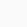# How to use greater than but not equivalent to(⋧) symbol in LaTeX?

Symbol/UnicodeGreater than but not equivalent to/U+22E7
Type of symbolMathematical Operators
Package (requirement)amssymb
ArgumentNo
Latex command\gnsim
Example\gnsim → ⋧

The best way to print the symbol in a latex document is to use the \gnsim command of the amssymb package. Below are some examples.

\documentclass{article}
\usepackage{amssymb}
\begin{document}
$ABC \gnsim \frac{2}{3}$
$XYZ \gnsim \frac{x+y}{2}$
$ABC \gnsim \frac{a+b}{c}$
\end{document}

Output :Scroll to Top